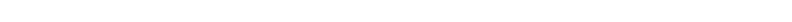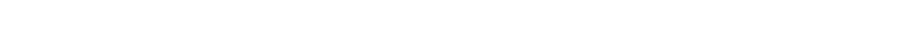For the Students of Hindu Vedic Astrology by Dr. A. Shanker

## Recent Posts## 20230607

Before determining the ascendant, it is necessary to correct the time. If the time on the watch is matching the railway time and lagan is established according to it there will be no need to make a double check. However, when there is no watch available and lagan has been established just by guess or the time in the watch is not accurate, it has to be rechecked for which we suggest some rules to facilitate those interested in astrology.

Rule 1: Accuracy of lagan and Sunrise and Sunset: watches run as per standard time, which differs in terms of Sunrise and Sunset as the Sun cannot keep the entire world lit up. Therefore, while establishing a lagan one should note that in case the lagan is determined as per standard watch there is no need to make adjustments to the time of birth. In case the lagan is determined by guessing how much of the day has worn up the time difference of 32 minutes should be added to or deducted from Madras time.

Rule 2: Conversion of time into pals: Convert the time passed from the Sunset till the required hour into ‘pal’ to be divided by 15. Add the numbers from Aries to Sun to the quotient. Again, divide the sum total by day 12. Count the remainder from the solar mansion (burj shamsi) onwards. The house on which the counting comes to an end will be the birth square. Hence, if the birth cell falls on the first or the ninth or fifth constellation of the zodiac from the ascendant cell the horoscope will be correct. Otherwise, it will be considered incorrect.

Rule 3: Quadruple the clocks, and if there are ‘pals’ add one if these are more than 15, 2 if more than 30, 3 if more than 45, and then count from the Aries mansion up to the Rashi where the Sun exists at that time add also the number thus obtained and let the sum total be divided by 12. Count the remaining number from the place where Sun is staying. The cell where that digit ends will be the birth cell. In case the birth cell falls in the first or the ninth or the fifth cell from the lagan the time of birth is correct. Otherwise, it will be incorrect. If the birth cell has been incorrectly established and one wants to correct it through this rule he will have to adjust the number of ‘ghari’ and ‘Pal’ of the required time. If a lesser time is guessed 30 pal are to be subtracted from the required time and in case a greater amount of time is guessed 30 pal will be added to the required time. Thus both the suspicious moment and time of birth will be corrected.

Rule 4: Suppose one’s time of birth is 13 ghari 48 pal. Now when the gharis will be quadrupled, we get 52 pals while the normal total number of pal is 45. Per rule, when 3 is added to them the total becomes 55. Since the Sun falls in the horoscope in Sagittarius lagan in the seventh cell from the house of Aries the digit of 7 shall be further added to it. Now it will make 62. Now when 62 is divided by 12 remainders will be 2. When two steps are counted from the location of the Sun, the second column is of Scorpio, which is the fifth from the ascendant in the horoscope. It shows that the indication of birth falls in the fifth cell and, therefore time of birth and lagan are correct. Had there been any difference the time of birth would have been adjusted accordingly.

Rule 5: Our time of birth is 18 ghari and 58 pals. Now, as per the rule, when gharis are quadrupled we get 72. Since ‘pal’ is more than 48 we are required to add 3 to this to make a total of 75. As the Sun is located in the ninth cell on the question chart 9 has further added to it to make a sum total of 84 which is fully divided by 12. Now when the two cells are counted from the location of the Sun the 12th cell is found to be of Scorpio, which is eleventh from the ascendant cell which is not as per rules 1, 5 or 9. Therefore, it is proved that our this birth time is not correct.

Rule 6: Since the result entirely depends on the accuracy of the time of birth and the fortunate solar cells and other cells are counted from the fortunate cell due to which all remaining consequences are bound to the lagan cell it is necessary to bring exactitude to it. At the given birth time does not correspond to the ascendant 20 ‘pals’ are added to the birth time of 18 ghari and 48 pal and thus are obtained 19 ghari and 15 pals. As per rule, when they are quadrupled, we get 76 and since pals do not exceed 15 one number is further added to gharis to make it 77. Whereas Sun has fallen in the ninth cell from Aries the digit of 9 is further added to it to make the total 86.

2.           Now when we count two cells from the location of the Sun in the horoscope the second cell from the Sun’s location falls in the Capricorn solstice which is the cell of fortune viz auspicious moment. It shows that the indication of birth occurs in the first cell. Therefore, both the time of birth and the ascendant are correct. Now all results will be correct. It there is any doubt about the stipulated volume of time let it be reduced a little to correct it.

Rule 7: The lunar mansion or the cell where the Moon is lying and where the lord of that mansion is staying will have a ‘paranpad’ in the third or the fifth or the ninth or eleventh cell from there. Now it is to be seen whether the cell containing the ‘paranpad’ digit is the third, the fifth, the ninth or the eleventh of it or not. It the cells has come to the third or the fifth, or the ninth or the eleventh from where the lord of that mansion stays the ascendant and the birth will be considered correct. Otherwise, it will be wrong.

Note: Enquire from three persons the estimated number of ‘gharis’ adds together the number told by them and divide the sum total by three. The quotient will be considered to be the required time.

Rule 8: When needed, whether it is day or might just, observe the breath rate. If the right tune is flowing there the odd ascendant will be in Aries, Gemini, Leo, Sagittarius or Aquarius. If the breath is flowing in the left turn the even ascendant will be in Taurus, Cancer, Virgo, Scorpio, Capricorn, or Pisces.

Rule 9: To the extent the digit of the ascendant is enumerable, count that ascendant digit along with the date, time, day and lunar mansion. The ascendant is represented by Aries, date by, first, day by Sunday and the lunar mansion by Ashni. Add 7 to the total number counted and divided the sum total by 5. One should suppose a yellow-colored object if the remainder is 1, a white-colored object if the remainder is 2, a red-colored object if the remainder is 3, a green-colored object if the remainder is 4 and a black-colored object if the sum total is fully divided. Let the inquirer just mention a color. If he depicts the color according to the remaining numbers the ascendant is correct. Otherwise, it will be wrong.## Astro Categories